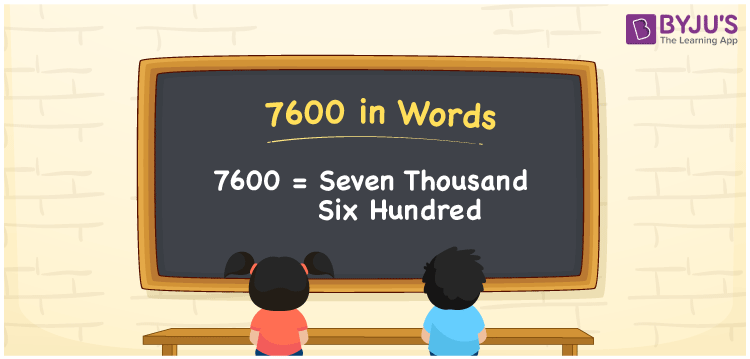# 7600 in Words

7600 in words is written as Seven thousand six hundred. We know that 7600 is a cardinal number as it denotes a specific amount or quantity. For example, a water dispenser is selling for Rs. 7600 in a shop; then you can convey the same to others as “A water dispenser is available at Seven thousand six hundred rupees at the shop”. In the same way, we can use the word form of the number 7600 in many scenarios.

 7600 in words Seven thousand six hundred Seven thousand six hundred in Numbers 7600

## 7600 in English Words

We generally write numbers in words with the help of the English alphabet. Thus, we can spell 7600 in English as “Seven thousand six hundred”.## How to Write 7600 in Words?

Let’s create a place value chart with four columns as given below since the number 7600 contains four digits.

 Thousands Hundreds Tens Ones 7 6 0 0

Here, ones = 0, tens = 0, hundreds = 6, and thousands = 7.

Thus, we can write the expanded form as given below.

7 × Thousand + 6 × Hundred + 0 × Ten + 0 × One

= 7 × 1000 + 6 × 100 + 0 × 10 + 0 × 1

= 7000 + 600

= Seven thousand + Six hundred

= Seven thousand six hundred

Hence, 7600 in words is written as Seven thousand six hundred.

As we know, 7600 is a natural number that precedes 7601 and succeeds 7599.

7600 in words – Seven thousand six hundred

Is 7600 an odd number? – No

Is 7600 an even number? – Yes

Is 7600 a prime number? – No

Is 7600 a composite number? – Yes

Is 7600 a perfect square number? – No

Is 7600 a perfect cube number? – No

## Frequently Asked Questions on 7600 in Words

Q1

### How do you write 7600 in words?

We can write the number 7600 in words as Seven thousand six hundred.
Q2

### What is the value of seven thousand six hundred minus seven thousand two hundred?

Seven thousand six hundred minus seven thousand two hundred = 7600 – 7200 = 400 Therefore, the value of seven thousand six hundred minus seven thousand two hundred is four hundred.
Q3

### How to write Rs. 7600 in words on a cheque?

On a cheque, we generally write the amount of Rs. 7600 in words as Seven thousand six hundred rupees only.